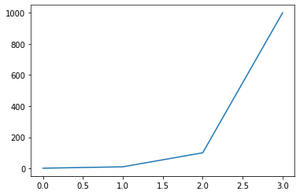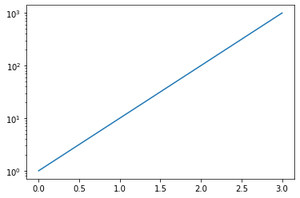Related Articles

# How to put the y-axis in logarithmic scale with Matplotlib ?

• Difficulty Level : Medium
• Last Updated : 17 Dec, 2020

Axes’ in all plots using Matplotlib are linear by default, yscale() method of the matplotlib.pyplot library can be used to change the y-axis scale to logarithmic.

The method yscale() takes a single value as a parameter which is the type of conversion of the scale, to convert y-axes to logarithmic scale we pass the “log” keyword or the matplotlib.scale.LogScale class to the yscale method.

Syntax :  matplotlib.pyplot.yscale(value, **kwargs)

Parameters:

• Value = { “linear”, “log”, “symlog”, “logit”, … }
• **kwargs = Different keyword arguments are accepted, depending on the scale (matplotlib.scale.LinearScale, LogScale, SymmetricalLogScale, LogitScale)

Returns : Converts the y-axes to the given scale type. (Here we use the “log” scale type)

Linear Scale Example :

## Python3

 `import` `matplotlib.pyplot as plt`` ` `data ``=` `[``10``*``*``i ``for` `i ``in` `range``(``4``)]``plt.plot(data)`

Output:Linear Scale

Logarithmic Scale Example :

## Python3

 `import` `matplotlib.pyplot as plt`` ` `data ``=` `[``10``*``*``i ``for` `i ``in` `range``(``4``)]`` ` `# convert y-axis to Logarithmic scale``plt.yscale(``"log"``)  ``plt.plot(data)`

Output:Logarithmic y-axis

Attention geek! Strengthen your foundations with the Python Programming Foundation Course and learn the basics.

To begin with, your interview preparations Enhance your Data Structures concepts with the Python DS Course. And to begin with your Machine Learning Journey, join the Machine Learning – Basic Level Course

My Personal Notes arrow_drop_up